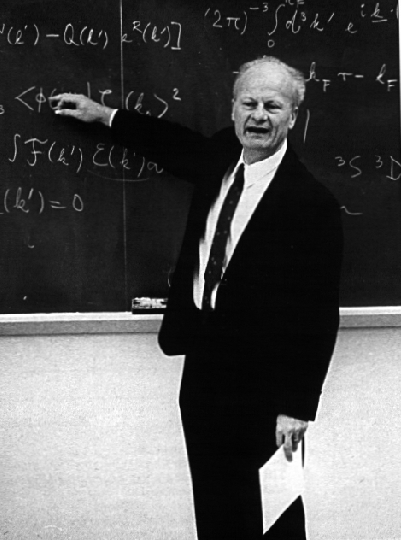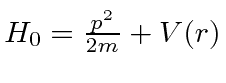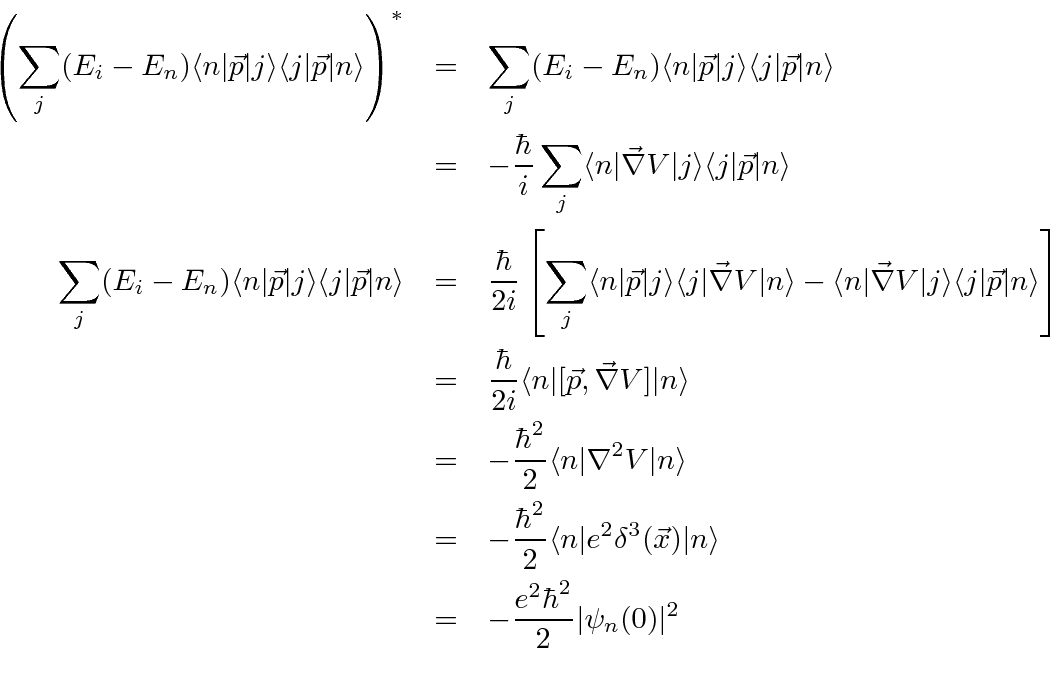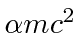## The Lamb Shift

In 1947, Willis E. Lamb and R. C. Retherford used microwave techniques to determine the splitting between theandstates in Hydrogen to have a frequency of 1.06 GHz, (a wavelength of about 30 cm). (The shift is now accurately measured to be 1057.864 MHz.) This is about the same size as the hyperfine splitting of the ground state.The technique used was quite interesting. They made a beam of Hydrogen atoms in thestate, which has a very long lifetime because of selection rules. Microwave radiation with a (fixed) frequency of 2395 MHz was used to cause transitions to thestate and a magnetic field was adjusted to shift the energy of the states until the rate was largest. The decay of thestate to the ground state was observed to determine the transition rate. From this, they were able to deduce the shift between theandstates.

Hans Bethe used non-relativistic quantum mechanics to calculate the self-energy correction to account for this observation.We now can compute the correction the same way he did.It is now necessary to discuss approximations needed to complete this calculation. In particular, the electric dipole approximation will be of great help, however, it is certainly not warranted for large photon energies. For a good E1 approximation we needeV. On the other hand, we want the cut-off for the calculation to be of order. We will use the E1 approximation and the high cut-off, as Bethe did, to get the right answer. At the end, the result from a relativistic calculation can be tacked on to show why it turns out to be the right answer. (We aren't aiming for the worlds best calculation anyway.)The log term varies more slowly than does the rest of the terms in the sum. We can approximate it by an average. Bethe used numerical calculations to determine that the effective average ofis. We will do the same and pull the log term out as a constant.This sum can now be reduced further to a simple expression proportional to theusing a typical clever quantum mechanics calculation. The basic Hamiltonian for the Hydrogen atom is.This must be a real number so we may use its complex conjugate.Only the s states will have a non-vanishing probability to be at the origin withand. Therefore, only the s states will shift in energy appreciably. The shift will be.This agrees far too well with the measurement, considering the approximations made and the dependence on the cut-off. There is, however, justification in the relativistic calculation. Typically, the full calculation was made by using this non-relativistic approach up to some energy of the order of, and using the relativistic calculation above that. The relativistic free electron self-energy correction diverges only logarithmically and a very high cutoff can be usedwithout a problem. The mass of the electron is renormalized as above. The Lamb shift does not depend on the cutoff and hence it is well calculated. We only need the non-relativistic part of the calculation up to photon energies for which the E1 approximation is OK. The relativistic part of the calculation down toyields.The non-relativistic calculation gave.So the sum of the two gives.The dependence oncancels. In this calculation, thein the log is the outcome of the relativistic calculation, not the cutoff. The electric dipole approximation is even pretty good since we did not need to go up to large photon energies non-relativistically and no E1 approximation is needed for the relativistic part. That's how we (and Bethe) got about the right answer.

The Lamb shift splits theandstates which are otherwise degenerate. Its origin is purely from field theory. The experimental measurement of the Lamb shift stimulated theorists to develop Quantum ElectroDynamics. The correction increases the energy of s states. One may think of the physical origin as the electron becoming less pointlike as virtual photons are emitted and reabsorbed. Spreading the electron out a bit decreases the effect of being in the deepest part of the potential, right at the origin. Based on the energy shift, I estimate that the electron in the 2s state is spread out by about 0.005 Angstroms, much more than the size of the nucleus.

The anomalous magnetic moment of the electron,, which can also be calculated in field theory, makes a small contribution to the Lamb shift.

Jim Branson 2013-04-22